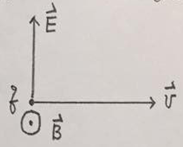### How long will it take for the ball to reach to top

Assignment Help Physics
##### Reference no: EM13722145

Question 1: A tennis ball is thrown straight up with initial velocity Vo. Assuming that the air drag on the ball varies with velocity as cv2, (c >0) where c is the coefficient of friction.

(a) How high will the tennis ball go?

(b) How long will it take for the ball to reach to top?

(c) How long will it take to return back to the ground?

(d) What is the final (impact) velocity when it reaches the ground? If you cannot get analytical expressions, try to get them numerically (Use Maple), let m=0.3 Kg, vo = 25 m/sec, and c = 0.05 Kg/m. Or derive the formulas. You must check your results for the frictionless limit.

Question 2: A particle of mass m is released from rest a distance b from a fixed origin of force that attracts according to the inverse cubic law, F(x) = -k/x2. How long will it take for the particle to reach the origin and what is the final velocity?

Question 3: A velocity selector is a device used to select charge q (assume positive for simplicity) particles with ironer speed v . Assume the following configuration of static electric E and B, (a) Derive the trajectory of the charge particles and (b) the condition that it will go straight without deflection.### Write a Review